# PSEB 12th Class Physics Solutions Chapter 4 Moving Charges and Magnetism

Punjab State Board PSEB 12th Class Physics Book Solutions Chapter 4 Moving Charges and Magnetism Textbook Exercise Questions and Answers.

## PSEB Solutions for Class 12 Physics Chapter 4 Moving Charges and Magnetism

PSEB 12th Class Physics Guide Moving Charges and Magnetism Textbook Questions and Answers

Question 1.
A circular coil of wire consisting of 100 turns, each of radius
8.0 cm carries a current of 0.40 A. What is the magnitude of the magnetic field B at the centre of the coil?
Number of turns on the circular coil, n = 100
Radius of each turn, r = 8.0 cm = 0.08 m
Current flowing in the coil, I = 0.4 A
Magnitude of the magnetic field at the centre of the coil is given by the relation,
$$|B|=\frac{\mu_{0}}{4 \pi} \frac{2 \pi n I}{r}$$
where, μ0 = 4π × 10-7 TmA-1
$$|B|$$ = $$\frac{4 \pi \times 10^{-7}}{4 \pi}$$ × $$\frac{2 \pi \times 100 \times 0.4}{0.08}$$
= 3.14 × 10-4T
Hence, the magnitude of the magnetic field is 3.14 × 10-4 T

Question 2.
A long straight wire carries a current of 35 A. What is the magnitude of the field B at a point 20 cm from the wire?
Current in the wire, I = 35 A
Distance of the point from the wire, r = 20 cm = 0.2 m
Magnitude of the magnetic field at this point is given as
B = $$\frac{\mu_{0}}{4 \pi} \frac{2 I}{r}$$
B = $$\frac{4 \pi \times 10^{-7} \times 2 \times 35}{4 \pi \times 0.2}$$
= 3.5 × 10-5T
Hence, the magnitude of the magnetic field at a point 20 cm from the wire is 3.5 × 10-5 T.Question 3.
A long straight wire in the horizontal plane carries a current of 50 A in north to south direction. Give the magnitude and direction of B at a point 2.5 m east of the wire.
Current in the wire, I = 50 A
A point is 2.5 m away from the east of the wire.
∴ Magnitude of the distance of the point from the wire, r = 2.5 m
Moving Charges and Magnetism ini
Magnitude of the magnetic field at that point is given by the relation,
B = $$\frac{\mu_{0} 2 I}{4 \pi r}$$
= $$\frac{4 \pi \times 10^{-7} \times 2 \times 50}{4 \pi \times 2.5}$$
= 4 × 10-6 T
The point is located normal to the wire length at a distance of 2.5 m. The direction of the current in the wire is vertically downward. Hence, according to the Maxwell’s right hand thumb rule, the direction of the magnetic field at the given point is vertically upward.

Question 4.
A horizontal overhead power line carries a current of 90 A in east to west direction. What is the magnitude and direction of the magnetic field due to the current 1.5 m below the line?
Current in the power line, I = 90 A
Point is located below the power line at distance, r = 1.5 m
Hence, magnetic field at that point is given by the relation,
B = $$\frac{\mu_{0} 2 I}{4 \pi r}$$
= $$\frac{4 \pi \times 10^{-7} \times 2 \times 90}{4 \pi \times 1.5}$$ = 1.2 × 10-5T
The current is flowing from east to west. The point is below the power line. Hence, according to Maxwell’s right hand thumb rule, the direction of the magnetic field is towards the south.

Question 5.
What is the magnitude of magnetic force per unit length on a wire carrying a current of 8 A and making an angle of 30° with the direction of a uniform magnetic field of 0.15 T?
Current in the wire, I = 8 A
Magnitude of the uniform magnetic field, B = 0.15 T
Angle between the wire and magnetic field, θ = 30°.
Magnetic force per unit length on the wire is given as,
F = BI sinθ
= 0.15 × 8 × sin30°
= 0.15 × 8 × $$\frac{1}{2}$$
= 0.15 × 4 = 0.6 Nm-1
Hence, the magnetic force per unit length on the wire is 0.6 Nm-1.Question 6.
A 3.0 cm wire carrying a current of 10 A is placed inside a solenoid perpendicular to its axis. The magnetic field inside the solenoid is given to be 0.27 T. What is the magnetic force on the wire?
Length of the wire, l = 3 cm = 0.03 m
Current flowing in the wire, I = 10 A
Magnetic field, B = 0.27 T
Angle between the current and magnetic field, θ = 90°
Magnetic force exerted on the wire is given as,
F = BIl sinθ
= 0.27 × 10 × 0.03 × sin 90°
= 8.1 × 10-2N
Hence, the magnetic force on the wire is 8.1 × 10-2 N.

Question 7.
Two long and parallel straight wires A and B carrying currents of
8.0 A and 5.0 A in the same direction are separated by a distance of 4.0 cm. Estimate the force on a 10 cm section of wire A.
Here, let I1 and I2 be the currents flowing in the straight long and parallel wires A and B respectively.
∴ I1 = 8.0 A, I2 = 5.0 A flowing in the same direction
r = distance between A and B = 4.0 cm = 4 × 10-2 m
If F’ be the force per unit length on wire A, then using
F’ = $$\frac{\mu_{0}}{4 \pi} \cdot \frac{2 I_{1} I_{2}}{r}$$, we get
F’ = 10-7 × $$\frac{2 \times 8 \times 5}{4 \times 10^{-2}}$$ Nm-1
= 20 × 10-5 Nm-1

If F be the force on a section of length 10 cm of wire A, then
F = F’ × l (Here,l = 10 × 10-2m)
= 20 × 10-5 × 10 × 10-2N
= 2 × 10-5N

Question 8.
A closely wound solenoid 80 cm long has 5 layers of windings of 400 turns each. The diameter of the solenoid is 1.8 cm. If the current carried is 8.0 A, estimate the magnitude of B inside the solenoid near its centre.
Length of the solenoid, l = 80 cm = 0.8 m
Number of turns in each layer = 400
Number of layers in the solenoid = 5
∴ Total number of turns on the solenoid, N = 5 × 400 = 2000
Diameter of the solenoid, D = 1.8 cm = 0.018 m
Current carried by the solenoid, I = 8.0 A
Magnitude of the magnetic field inside the solenoid near its centre is given by the relation,
g.hoM
B = $$=\frac{\mu_{0} N I}{l}$$
B = $$\frac{4 \pi \times 10^{-7} \times 2000 \times 8}{0.8}$$
= 8 π × 10-3 = 2.512 × 10-2 T
Hence, the magnitude of the magnetic field inside the solenoid near its centre is 2.512 × 10-2 T.Question 9.
A square coil of side 10 cm consists of 20 turns and carries a current of 12 A. The coil is suspended vertically and the normal to the plane of the coil makes an angle of 30° with the direction of a uniform horizontal magnetic field of magnitude 0.80 T. What is the magnitude of torque experienced by the coil?
Length of a side of the square coil, l = 10 cm = 0.1 m
Current flowing in the coil, I = 12 A
Number of turns on the coil, N = 20
Angle made by the plane of the coil with magnetic field, θ = 30°
Strength of magnetic field, B = 0.80 T
Magnitude of the torque experienced by the coil in the magnetic field is given by the relation,
τ = NBIAsinθ
where, A = Area of the square coil
⇒ l × l = 0.1 × 0.1 = 0.01 m2
∴ τ = 20 × 0.80 × 12 × 0.01 × sin30°
= 20 × 0.80 × 12 × 0.01 × $$\frac{1}{2}$$
= 0.96 N m
Hence, the magnitude of the torque experienced by the coil is 0.96 N m.

Question 10.
Two moving coil meters, M1 and M2 have the following particulars:
R1 = 10 Ω, N1 = 30,
A1 = 3.6 × 10-3 m2, B1 = 0.25T
R2 = 14Ω, N2 = 42,
A2 = 1.8 × 10-3 m2, B2 = 0.50 T
(The spring constants are identical for the two meters). Determine the ratio of (a) current sensitivity and (b) voltage sensitivity of M2 and M1.
Here,R1 = 10 n, N1 = 30, A1 = 3.6 x 10-3 m2,B1 = 0.25T for coil M1
R2 = 14 Q, N2 = 42, A2 = 1.8 x 10-3 m2,B2 = 0.50T for coil M2.
We know that current sensitivity and voltage sensitivity are given by the formulae
Current sensitivity = $$\frac{N B A}{k}$$
and Voltage sensitivity = $$\frac{N B A}{k R}$$
Here, k1 = k2 for the two coils = k (say)
∴ Current sensitivity for M1 is given by = N1B1A1/ k and for M2 = N2B2A2 / k

(a) Current sensitivity ratio for M2 and M1 is given by
= $$\frac{\frac{N_{2} B_{2} A_{2}}{k}}{\frac{N_{1} B_{1} A_{1}}{k}}$$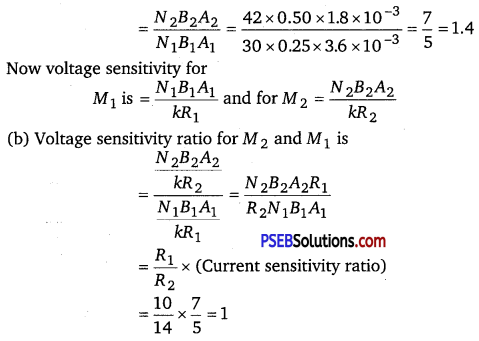Question 11.
In a chamber, a uniform magnetic field of 6.5 G (1 G = 10-4 T) is maintained. An electron is shot into the field with a speed of 4.8 × 106 ms-1 normal to the field. Explain why the path of the electron is a circle. Determine the radius of the circuit orbit.
e,= 1.6 × 10-19 (me = 9.1 × 10 -31 kg
Magnetic field strength, B = 6.5 G = 6.5 × 10-4 T
Speed of the electron, y = 4.8 × 106 m/s
Charge on the electron, e,= 1.6 × 10-19 C
Mass of the electron, me 9.1 × 10-31 kg
Angle between the electron and magnetic field, θ = 90°
Magnetic force exerted on the electron in the magnetic field is given as :
F = evBsinθ
This force provides centripetal force to the moving electron. Hence, the electron starts moving in a circular path of radius r.
Hence, centripetal force exerted on the electron,
Fe = $$\frac{m v^{2}}{r}$$
In equilibrium, the centripetal force exerted on the electron is equal to the magnetic force t.e.,
Fe = F
$$\frac{m v^{2}}{r}$$ = evBsinθ
r = $$\frac{m v}{B e \sin \theta}$$
= $$\frac{9.1 \times 10^{-31} \times 4.8 \times 10^{6}}{6.5 \times 10^{-4} \times 1.6 \times 10^{-19} \times \sin 90^{\circ}}$$
= 4.2 × 10-2 m = 4.2 cm
Hence, the radius of the circular orbit of the electron is 4.2 cm.Question 12.
In Exercise 4.11 obtain the frequency of revolution of the electron in its circular orbit. Does the answer depend on the speed of the electron? Explain.
Magnetic field strength, B = 6.5 × 10-4 T
Charge on the electron, e = 1.6 × 10-19 C
Mass of the electron, me = 9.1 × 10-31 kg
Velocity of the electron, v = 4.8 × 106 m/s
Radius of the orbit, r = 4.2 cm = 0.042 m
Frequency of revolution of the electron = v
Angular frequency of the electron = ω = 2πv
Velocity of the electron is related to the angular frequency as :
v = rω
In the circular orbit, the magnetic force on the electron is balanced by the centripetal force. Hence, we can write :
evB = $$\frac{m v^{2}}{r}$$
eB = $$\frac{m}{r}$$ (rω) = $$\frac{m}{r}$$ (r2πv)
v = $$\frac{B e}{2 \pi m}$$

This expression for frequency is independent of the speed of the electron. On substituting the known values in this expression, we get the frequency as:
V = $$=\frac{6.5 \times 10^{-4} \times 1.6 \times 10^{-19}}{2 \times 3.14 \times 9.1 \times 10^{-31}}$$
= 18.2 × 106 Hz ≈ 18 MHz
Hence, the frequency of the electron is around 18 MHz and is independent of the speed of the electron.

Question 13.
(a) A circular coil of 30 turns and radius 8.0 cm carrying a current of 6.0 A is suspended vertically in a uniform horizontal magnetic field of magnitude 1.0 T. The field lines make an angle of 60° with the normal of the coil. Calculate the magnitude of the counter torque that must be applied to prevent the coil from turning.

(b) Would your answer change, if the circular coil in (a) were replaced by a planar coil of some irregular shape that encloses, the same area? (All other particulars are also unaltered.)
(a) Number of turns on the circular coil, N = 30
Radius of the coil, r = 8.0 cm = 0.08 m
Area of the coil = πr2 = π(0.08)2 = 0.0201 m2
Current flowing in the coil, I = 6.0 A
Magnetic field strength, B = 1.0 T
Angle between the field lines and normal with the coil surface,
θ = 60°
The coil experiences a torque in the magnetic field. Hence, it turns. The. counter torque applied to prevent the coil from turning is given by the relation,
τ = N IBAsinθ …………… (1)
= 30 × 6 × 1 × 0.0201 × sin60°
= 180 × 0.0201 × $$\frac{\sqrt{3}}{2}$$
= 3.133 Nm

(b) It can be inferred from relation (1) that the magnitude of the applied torque is not dependent on the shape of the coil. It depends on the area of the coil. Hence, the answer would not change if the circular coil in the above case is replaced by a planar coil of some irregular shape that encloses the same area.Question 14.
Two concentric circular coils X and Y of radii 16 cm and 10 cm, respectively, lie in the same vertical plane containing the north to south direction. Coil X has 20 turns and carries a current of 16 A; coil Y has 25 turns and carries a current of 18 A. The sense of the current in X is anticlockwise, and clockwise in Y, for an observer looking at the coils facing west. Give the magnitude and direction of the net magnetic field due to the coils at their centre.
Radius of coil X, r1 = 16 cm = 0.16 m
Radius of coil Y, r2 = 10 cm = 0.10 m
Number of turns on coil X, n1 = 20
Number of turns on coil Y, n2 = 25
Current in coil X,I1 =16 A
Current in coil Y, I2 = 18 A
Magnetic field due to coil X at their centre is given by the relation,
B1 = $$\frac{\mu_{0} n_{1} I_{1}}{2 r_{1}}$$
∴ B1 = $$\frac{4 \pi \times 10^{-7} \times 20 \times 16}{2 \times 0.16}$$
= 4π × 10-4 T (towards East)
Magnetic field due to coil Y at their centre is given by the relation,
B2 = $$\frac{\mu_{0} n_{2} I_{2}}{2 r_{2}}$$
$$\frac{4 \pi \times 10^{-7} \times 25 \times 18}{2 \times 0.10}$$
= 9π × 10-4 T (towards West)

Hence, net magnetic field can be obtained as:
B = B2 – B1
= 9π × 10-4 – 4π × 10-4
= 5π × 10 T
= 1.57 × 10-3 T (towards West)

Question 15.
A magnetic field of 100 G (1 G = 10-4 T) is required which is uniform in a region of linear dimension about 10 cm and area of cross-section about 10-3 m2. The maximum current-carrying capacity of a given coil of wire is 15 A and the number of turns per unit length that can be wound round a core is at most 1000 turns m-1 . Suggest some appropriate design particulars of a solenoid for the required purpose. Assume the core is not ferromagnetic.
Here, B = magnetic field = 100 G = 100 × 10-4 = 10-2 T,
Imax = maximum current carried by the coil = 15 A
n = number of turns per unit length = 1000 turns m-1 = 10 tums/cm
l = length of linear region = 10 cm
A = area of cross-section = 10-3 m2.

To produce a magnetic field in the above mentioned region, a solenoid can be made so that well within the solenoid, the magnetic field is uniform. To do so, we may take the length L of the solenoid 5 times the length of the region and area of the solenoid 5 times the area of region.

∴ L = 5l = 5 × 10 = 50 cm = 0.5m
and A = 5 × 10-3 m2
∴ If r be the radius of the solenoid, then
πr2 = A = 5 × 10-3
or r = $$\sqrt{\frac{5 \times 10^{-3}}{3.14}}$$ = 0.04 m = 4 cm
Also let us wind 500 turns on the coil so that the number of turns per m is
n = $$\frac{500}{0.5}$$ = 1000 turns m-1
∴ Using formula, μ0nI = B, we get
I = $$\frac{B}{\mu_{0} n}$$ = $$\frac{10^{-2}}{4 \pi \times 10^{-7} \times 1000}$$ = 7.96 A ≈ 8A

So, a current of 8 A can be passed through it to produce a uniform magnetic field of 100 G in the region. But this is not a unique way. If we wind 300 turns on the solenoid, then number of turns is
n = $$\frac{300}{0.5}$$ = 600 per m.
∴ I= $$\frac{B}{\mu_{0} n}$$ = $$\frac{10^{-2}}{4 \pi \times 10^{-7} \times 600}$$ = 13.3 A
i. e., a current of 13.3 A can be passed through it to produce the magnetic field of loo G.
Similarly, if no. of turns = 400,
then, n = $$\frac{400}{0.5}$$ = 800 per m.
∴ I = $$\frac{B}{\mu_{0} n}$$ = $$\frac{10^{-2}}{4 \pi \times 10^{-7} \times 800}$$ = 9.95 A
i. e., a current of 10 A can be passed ≈ 10 A
Through it to produce B = 100 G
Thus we may achieve the result in a number of ways.Question 16.
For a circular coil of radius R and N turns carrying current J, the magnitude of the magnetic field at a point on its axis at a distance x from its centre is given by,
B = $$\frac{\mu_{0} I R^{2} N}{2\left(x^{2}+R^{2}\right)^{3 / 2}}$$
(a) Show that this reduces to the familiar result for field at the centre of the coil.
(b) Consider two parallel co-axial circular coils of equal radius R, and number of turns N, carrying equal currents in the same direction, and separated by a distance R. Show that the field on the axis around the mid-point between the coils is uniform over a distance that is small as compared to JR, and is given by,
B = 0.72 $$\frac{\mu_{0} \boldsymbol{N I}}{\boldsymbol{R}}$$, approximately.
[Such an arrangement to produce a nearly uniform magnetic field over a small region is known as Helmholtz coils.]
Radius of circular coil = R
Number of turns on the coil = N
Current in the coil = I
Magnetic field at a point on its axis at distance x from its centre is given by the relation,
B = $$\frac{\mu_{0} I R^{2} N}{2\left(x^{2}+R^{2}\right)^{3 / 2}}$$

(a) If the magnetic field at the centre of the coil is considered, then x = 0
∴ B = $$\frac{\mu_{0} I R^{2} N}{2 R^{3}}=\frac{\mu_{0} I N}{2 R}$$
This is the familiar result for magnetic field at the centre of the coil,

(b) Radii of two parallel co-axial circular coils = R
Number of turns on each coil = N
Current in both coils = I
Distance between both the coils = R
Let us consider point Q at distance d from the centre.
Then, one coil is at a distance of $$\frac{R}{2}$$ + d from point Q.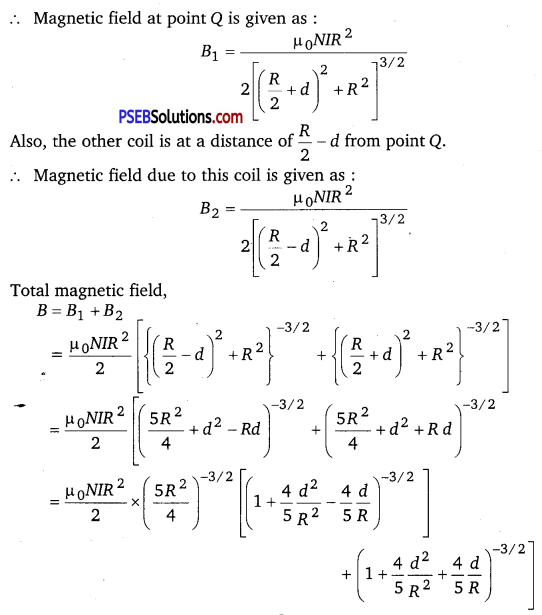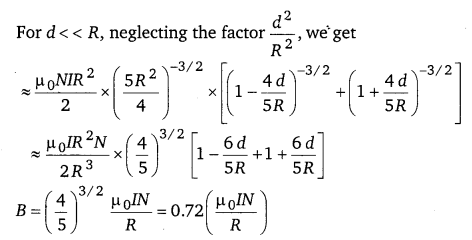Hence, it is proved that the field on the axis around the mid-point between the coils is uniform.

Question 17.
A toroid has a core (non-ferromagnetic) of inner radius 25 cm and outer radius 26 cm, around which 3500 turns of a wire are wound. If the current in the wire is 11 A, what is the magnetic field (a) outside the toroid, (b) inside the core of the toroid, and (c) in the empty space surrounded by the toroid.
Here, I = 11 A,
Total number of turns = 3500
Mean radius of toroid, r = $$\frac{25+26}{2}$$
r = 25.5cm = 25.5 × 10-2 m
Total length of the toroid = 2πr = 2π × 25.5 × 10-2
= 51π × 10-2m
Therefore, number of turns per unit length,
n = $$\frac{3500}{51 \pi \times 10^{-2}}$$

(a) The field is non-zero only inside the core surrounded by the windings of the toroid. Therefore, the field outside the toroid is zero.

(b) The field inside the core of the toroid
B = μ0nI
B = 4π × 10-7 × $$\frac{3500}{51 \pi \times 10^{-2}}$$ × 11
B = 3.02 × 10-2 T

(c) For the reason given in (a), the field in the empty space surrounded by toroid is also zero.Question 18.
(a) A magnetic field that varies in magnitude from point to point but has a constant direction (east to west) is set up in a chamber. A charged particle enters the chamber and travels undeflected along a straight path with constant speed. What can you say about the initial velocity of the particle?

(b) A charged particle enters an environment of a strong and non-uniform magnetic field varying from point to point both in magnitude and direction and comes out of it following a complicated trajectory. Would its final speed equal the initial speed if it suffered no collisions with the environment?

(c) An electron travelling west to east enters a chamber having a uniform electrostatic field in north to south direction. Specify the direction in which a uniform magnetic field should be set up to prevent the electron from deflecting from its straight line path.
(a) The initial velocity of the particle is either parallel or anti-parallel to the magnetic field. Hence, it travels along a straight path without suffering any deflection in the field.

(b) Yes, the final speed’ of the charged particle will be equal to its initial speed. This is because magnetic force can change the direction of velocity, but not its magnitude.

(c) An electron travelling from west to east enters a chamber having a uniform electrostatic field in the north-south direction. This moving electron can remain undeflected if the electric force acting on it is equal and opposite of magnetic field. Magnetic force is directed towards the south. According to Fleming’s left hand rule, magnetic field should be applied in a vertically downward direction.

Question 19.
An electron emitted by a heated cathode and accelerated through a potential difference of 2.0 kV, enters a region with uniform magnetic field of 0.15 T. Determine the trajectory of the electron if the field (a) is transverse to its initial velocity, (b) makes an angle of 30° with the initial velocity.
Magnetic field strength, B = 0.15 T
Charge on the electron, e = 1.6 × 10-19C
Mass of the electron, m = 9.1 × 10-31 kg
Potential difference, V = 2.0 kV = 2 × 103 V
Thus, kinetic energy of the electron = eV
⇒ eV = $$\frac{1}{2}$$mv2
v = $$\sqrt{\frac{2 e V}{m}}$$ ……………. (1)
where, v = Velocity of the electron
Magnetic force on the electron provides the required centripetal force of the electron. Hence, the electron traces a circular path of radius r.

(a) When the magnetic field is transverse to the initial velocity. The force on the electron due to transverse magnetic field = Bev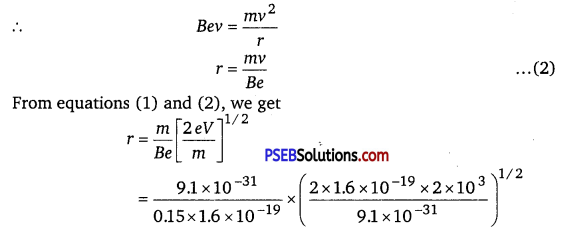= 100.55 × 10-5
= 1.01 × 10-3 m = 1 mm
Hence, the electron has a circular trajectory of radius 1.0 mm normal to the magnetic field.

(b) When the magnetic field makes an angle θ of 30° with initial velocity, the initial velocity will be,
v1 = vsinθ
From equation (2), we can write the expression for new radius as :
r1 = $$\frac{m v_{1}}{B e}$$
= $$\frac{m v \sin \theta}{B e}$$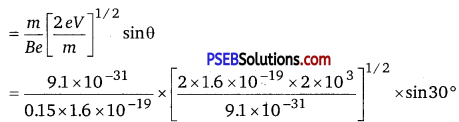= 0.5 × 10-3 m = 0.5mm
Hence, the electron has a helical trajectory of radius 0.5 mm along the magnetic field direction.Question 20.
A magnetic field set up using Helmholtz coils (described in Exercise 4.16) is uniform in a small region and has a magnitude of 0.75 T. In the same region, a uniform electrostatic field is maintained in a direction normal to the common axis of the coils. A narrow beam of (single species) charged particles all accelerated through 15 kV enters this region in a direction perpendicular to both the axis of the coils and the electrostatic Held. If the beam remains undeflected when the electrostatic field is 9.0 × 10-5 V m-1, make a simple guess as to what the beam contains. Why is the answer not unique?
Magnetic field, B = 0.75 T
Accelerating voltage, V = 15 kV = 15 × 103 V
Electrostatic field, E = 9 × 10-5 Vm-1
Mass of the electron = m
Charge on the electron = e
Velocity of the electron = v
Kinetic energy of the electron = eV
⇒ $$\frac{1}{2}$$mv2 = eV
∴ $$\frac{e}{m}=\frac{v^{2}}{2 V}$$ ……………. (1)
Since the particle remains undeflected by electric and magnetic fields, we can infer that the electric field is balancing the magnetic field.
∴ eE = evB
v = $$\frac{E}{B}$$ …………. (2)
Putting equation (2) in equation (1), we get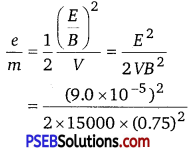= 4.8 × 107 C/kg
Also, we know that $$\frac{e}{m}$$ for proton is 9.6 × 10-7 C kg-1 . It follows that the charged particle under reference has the value of $$\frac{e}{m}$$ half of that for the
proton, so its mass is clearly double the mass of proton. Thus the beam may be of deutrons.
The answer is not unique as the ratio of charge to mass i. e.,
4.8 × 107 C kg-1 may be satisfied by many other charged particles, surch as
He++($$\frac{2 e}{2 m}$$) and Li3+ ($$\frac{3 e}{3 m}$$)
which have the same value of $$\frac{e}{m}$$.

Question 21.
A straight horizontal conducting rod of length 0.45 m and mass 60 g is suspended by two vertical wires at its ends. A current of 5.0 A is set up in the rod through the wires.
(a) What magnetic field should be set up normal to the conductor in order that the tension in the wires is zero?
(b) What will be the total tension in the wires if the direction of current is reversed keeping the magnetic field same as before? (Ignore the mass of the wires.) g = 9.8 ms-2.
Length of the rod, l = 0.45 m
Mass of the rod, m = 60 g = 60 × 10-3 kg
Acceleration due to gravity, g = 9.8 m/s2
Current in the rod flowing through the wire, I = 5 A

(a) Magnetic field (B) is equal and opposite to the weight of the rod i.e.,
BIl = mg
∴ B = $$\frac{m g}{I l}$$ = $$\frac{60 \times 10^{-3} \times 9.8}{5 \times 0.45}$$ = 0.26T
A horizontal magnetic field of 0.26 T normal to the length of the conductor should be set up in order to get zero tension in the wire. The magnetic field should be such that Fleming’s left hand rule gives an upward magnetic force.

(b) If the direction of the current is reversed, then the force due to magnetic field and the weight of the rod acts in a vertically downward direction.
∴ Total tension in the wire = BIl + mg
= 0.26 × 5 × 0.45 + (60 × 10-3) × 9.8
= 1.176 NQuestion 22.
The wires which connect the battery of an automobile to its starting motor carry a current of 300 A (for a short time). What is the force per unit length between the wires if they are 70 cm long and 1.5 cm apart? Is the force attractive or repulsive?
Current in both wires, I = 300 A
Distance between the wires, r = 1.5 cm = 0.015 m
Length of the both wires, l = 70 cm = 0.7 m
Force between the two wires is given by the relation,
F = $$\frac{\mu_{0} I^{2}}{2 \pi r}$$
∴ F = $$\frac{4 \pi \times 10^{-7} \times(300)^{2}}{2 \pi \times 0.015}$$ = 1.2 N/M
Since the direction of the current in the wires is opposite, a repulsive force exists between them.

Question 23.
A uniform magnetic field of 1.5 T exists in a cylindrical region of radius 10.0 cm, its direction parallel to the axis along east to west. A wire carrying current of 7.0 A in the north to south direction passes through this region. What is the magnitude and direction of the force on the wire if,
(a) the wire intersects the axis,
(b) the wire is turned from N-S to northeast-northwest direction,
(c) the wire in the N-S direction is lowered from the axis by a distance of 6.0 cm?
Magnetic field strength, B = 1.5 T
Radius of the cylindrical region, r = 10cm = 0.1m
Current in the wire passing through the cylindrical region, I = 7 A

(a) If the wire intersects the axis, then the length of the wire is the diameter of the cylindrical region.
Thus, l = 2r = 2 × 0.1 = 0.2 m
Angle between magnetic field and current, θ = 90°
Magnetic force acting on the wire is given by the relation,
F = BIl sinθ
= 1.5 × 7 × 0.2 × sin90° = 2.1N
Hence, a force of 2.1 N acts on the wire in a vertically downward direction.

(b) New length of the wire after turning it to the northeast-northwest direction can be given as:
l1 = $$\frac{l}{\sin \theta}$$
Angle between magnetic field and current, θ = 45°
Force on the wire,
F1 = BIl1 sinθ
$$\frac{B I l}{\sin \theta}$$ = sinθ
= BIl = 1.5 × 7 × 0.2 = 2.1 N
Hence, a force of 2.1 N acts vertically downward on the wire. This is independent of angle because Z sinG is fixed.

(c) The wire is lowered from the axis by distance, d = 6.0 cm
Let l2 be the new length of the wire.
∴ ($$\frac{l_{2}}{2}$$)2 = 4(d + r)
= 4 (10 + 6) = 4(16)
∴ l2 = 8 × 2 = 16 cm = 0.16 m
Magnetic force exerted on the wire,
F2 = BIl2
= 1.5 × 7 × 0.16 = 1.68 N
Hence, a force of 1.68 N acts in a vertically downward direction on the wire.Question 24.
A uniform magnetic field of 3000 G is established along the positive z-direction. A rectangular loop of sides 10 cm and 5 cm carries a current of 12 A. What is the torque on the loop in the different cases shown in Fig. 4.28? What is the force on each case? Which case corresponds to stable equilibrium?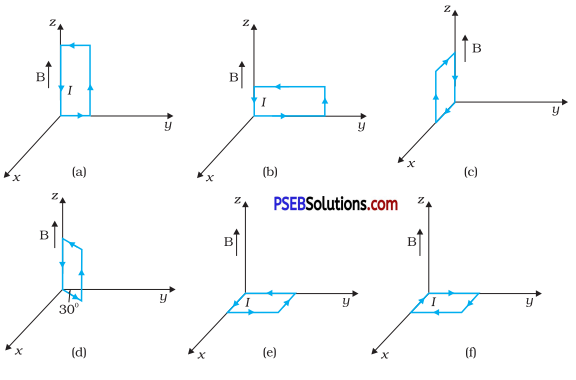Here,
B = uniform magnetic field
= 3000 gauss along z-axis
= 3000 × 10-4T = 0.3 T
l = length of rectangular loop
= 10 cm = 0.1 m
b = breath of rectangular loop
= 5 cm = 0.05 m
∴ A = area of rectangular loop
= l × b = 10 × 5 = 50cm2 = 50 × 10-4 m2
Torque on the loop is given by
$$\vec{\tau}$$ = (I$$\vec{A}$$) × $$\vec{B}$$
IA = 50 × 10-4 × 12 = 0.06 Am+2

(a) Here, I$$\vec{A}$$ = 0.06î Am2, $$\vec{B}$$ = 0.3k̂T
∴ $$\vec{\tau}$$ = 0.06î × 0.3k̂= -1.8 × 10-2 Nm ĵ
i.e., τ = 1.8 × 10-2 Nm and acts along negative y-axis.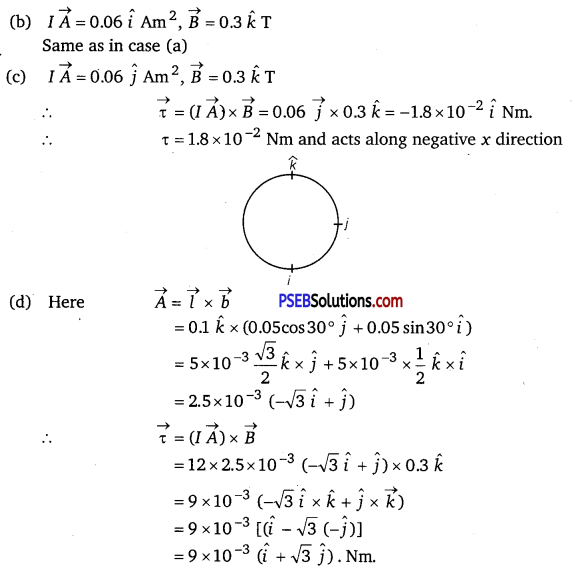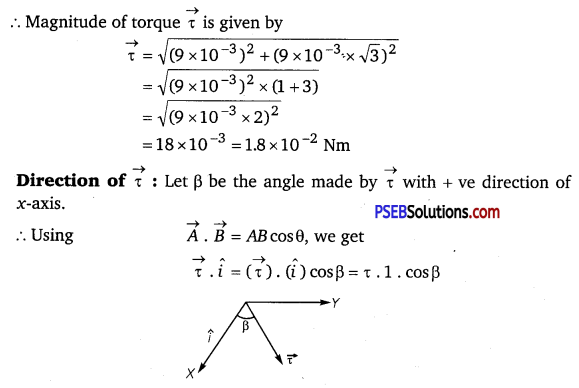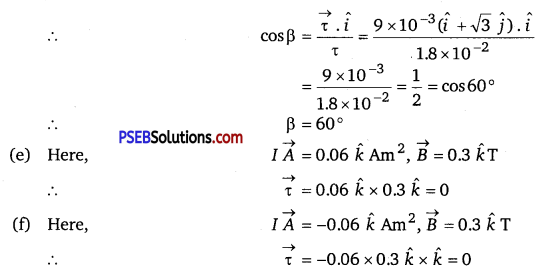Net force on a planar loop in a magnetic field is always zero, so force is
zero in each case.
Case (e) corresponds to stable equilibrium as 7 A is aligned with B while (f) corresponds to unstable equilibrium as 7 A is antiparallel to B.Question 25.
A circular coil of 20 turns and radius 10 cm is placed in a uniform magnetic field of 0.10 T normal to the plane of the coil. If the current in the coil is 5.0 A, what is the
(a) total torque on the coil,
(b) total force on the coil,
(c) average force on each electron in the coil due’ to the magnetic field?
(The coil is made of copper wire of cross-sectional area 10-5 m2, and the free electron density in copper is given to be about 1029 m-3.)
Number of turns on the circular coil, n = 20
Radius of the coil, r = 10cm = 0.1m
Magnetic field strength, B = 0.10 T
Current in the coil, I = 5.0 A
(a) The total torque on the coil is zero because the field is uniform.
(b) The total force on the coil is zero because the field is uniform.
(c) Cross-sectional area of copper coil, A = 10-5 m2
Number of free electrons per cubic meter in copper, N = 1029 / m3
Charge on the electron, e = 1.6 × 10-19C
Magnetic force, F = Bevd
Where, vd = $$\frac{I}{N e A}$$
∴ F = $$\frac{B e I}{N e A}=\frac{B I}{N A}$$ = $$\frac{0.10 \times 5.0}{10^{29} \times 10^{-5}}$$ 5 × 10-25N
Hence, the average force on each electron is 5 × 10-25 N.

Question 26.
A solenoid 60 cm long and of radius 4.0 cm has 3 layers of windings of 300 turns each. A 2.0 cm long wire of mass 2.5 g lies inside the solenoid (near its centre) normal to its axis; both the wire and the axis of the solenoid are in the horizontal plane. The wire is connected through two leads parallel to the axis of the solenoid to an external battery which supplies a current of 6.0 A in the wire. What value of current (with appropriate sense of circulation) in the windings of the solenoid can support the weight of the wire? g = 9.8 ms-2.
Length of the solenoid, L = 60 cm = 0.6 m
Radius of the solenoid, r = 4.0 cm = 0.04 m
It is given that there are 3 layers of windings of 300 turns each.
∴ Total number of turns, n = 3 × 300 = 900
Length of the wire, l = 2 cm = 0.02 m
Mass of the wire, m = 2.5 g = 2.5 × 10 -3 kg
Current flowing through the wire, i = 6 A
Acceleration due to gravity, g=9.8m/s2
Magnetic field produced inside the solenoid, B = $$\frac{\mu_{0} n I}{L}$$
where, μ0 = 4π × 10-7 TmA-1
I = Current flowing through the windings of the solenoid Magnetic force is given by the relation,
F = Bil = $$\frac{\mu_{0} n i I}{L}$$l
Also, the force on the wire is equal to the weight of the wire.
∴ mg = $$\frac{\mu_{0} n \text { Iil }}{L}$$
I = $$\frac{m g L}{\mu_{0} \text { nil }}$$
= $$\frac{2.5 \times 10^{-3} \times 9.8 \times 0.6}{4 \pi \times 10^{-7} \times 900 \times 0.02 \times 6}$$ = 108A
Hence, the current flowing through the solenoid is 108 A.Question 27.
A galvanometer coil has a resistance of 12 Ω and the metre shows full scale deflection for a current of 3 mA. How will you convert the metre into a voltmeter of range 0 to 18 V?
Resistance of the galvanometer coil, G = 12 Ω
Current for which there is full scale deflection, Ig = 3 mA = 3 × 10-3 A
Range of the voltmeter is 0, which needs to be converted to 18 V.
∴ V = 18 V
Let a resistor of resistance R be connected in series with the galvanometer to convert it into a voltmeter. This resistance is given as
R = $$\frac{V}{I_{g}}$$ – G
= $$\frac{18}{3 \times 10^{-3}}$$ – 12 = 6000 – 12 = 5988 Ω
Hence, a resistor of resistance 5988 Ω is to be connected in series with the galvanometer.

Question 28.
A galvanometer coil has a resistance of 15 Ω and the metre shows full scale deflection for a current of 4 mA. How will you convert the metre into an ammeter of range 0 to 6 A?
S = $$\frac{I_{g} G}{I-I_{g}}$$ = $$\frac{4 \times 10^{-3} \times 15}{6-4 \times 10^{-3}}$$
S = $$\frac{6 \times 10^{-2}}{6-0.004}=\frac{0.06}{5.996}$$ ≈ 0.01Ω = 10mΩ